# High School Physics : Understanding SI Units

## Example Questions

1 3 Next →

### Example Question #21 : High School Physics

The fastest time to finish a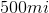driving race was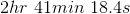. What was the average speed for the car during the race?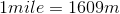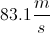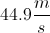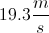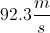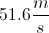Explanation:

This is a straightforward problem, but requires a number of unit conversions. To find the speed, we will need to determine the change in distance per unit time: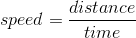While we are given distance and time, we need to convert it to meters per second. A distance of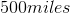is converted to meters with the relation.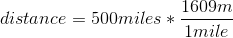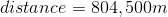Time requires several step-wise conversion. First, convert the hours to minutes.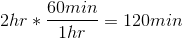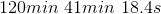Find the total number of minutes and convert it to seconds.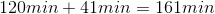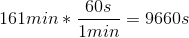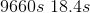Finally, find the total number of seconds.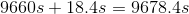Use the final distance and final time to calculate the speed in meters per second.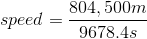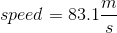### Example Question #21 : Understanding Si Units

Which of the following is the correct SI unit for distance?

Mile

Kilometer

Meter

Foot

Inch

Meter

Explanation:

First, when thinking about SI you should usually start with the metric system -- this leaves us with meter and kilometer. The correct SI unit for distance is the meter -- displacement is in meters, velocity is in meters per second, etc.

### Example Question #23 : High School Physics

Which of these is not an SI unit?

Kilometer

Second

Ohm

Tesla

Kilometer

Explanation:

While a kilometer is a metric unit of distance, the correct SI unit for distance is the meter. When making calculations with length, it is necessary to convert kilometers to meters.

Seconds are the SI unit for time. Ohms are the SI unit for electrical resistance. Farads are the SI unit for capacitance. Teslas are the SI unit for magnetic field strength.

### Example Question #24 : High School Physics

Which of the following is not an SI unit?

Meters

Decibels

Mass

Seconds